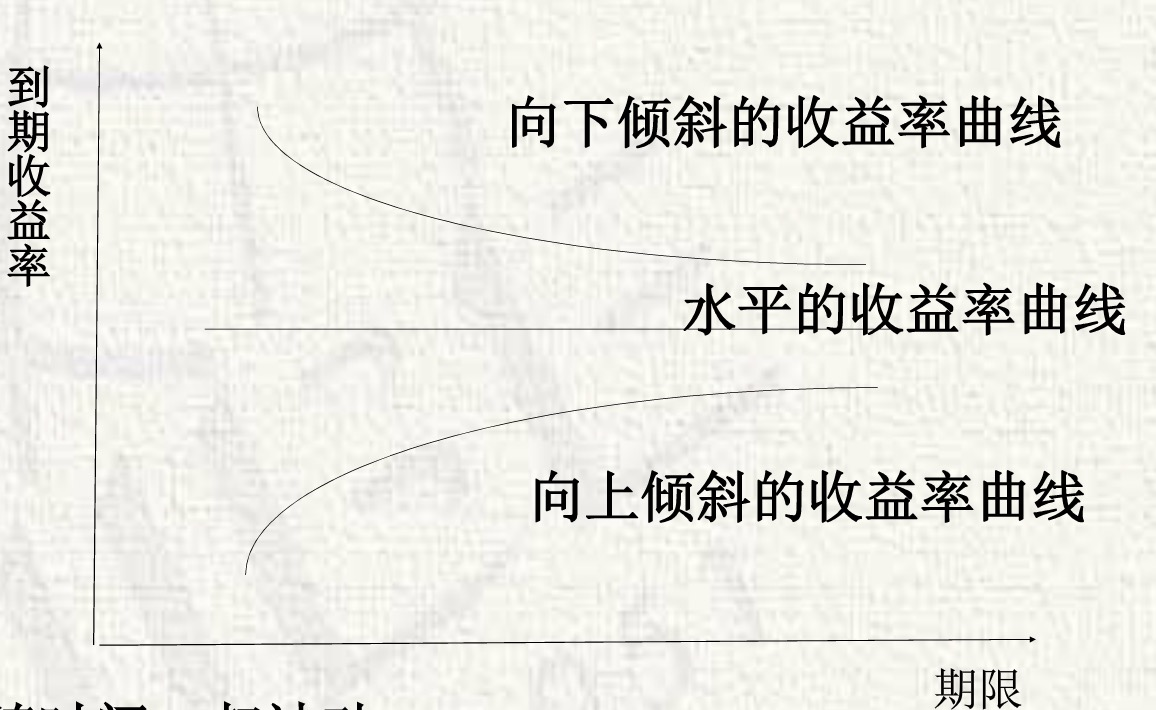# 利率(Interest rate)

Posted by GwanSiu on February 1, 2020

## 1. 利息(Interest)和利率(Interest Rate)

1. 什么是利率？

1.1.Interest rate(from wiki): an interest rate is the amount of interest due per period, as a proportion of the amount lent, deposited or borrowed (called the principal sum).

The concept of present value (or present discounted value) is based on the commonsense notion that a dollar paid to you one year from now is less than a dollar paid to you today.

1. 基准利率: 美国的联邦基金利率；中国的中央人民银行的存款贷款的基准利率；各国央行的贴现率。
1. 名义利率与实际利率 $\begin{equation} \text{实际利率=名义利率-通货膨胀率}. \end{equation}$

1. 年利率，又称年息: 百分之几(分)
2. 月利率，又称月息: 千分之几(厘)
3. 日利率，又称日息: 万分之几(毫)

## 2. 利息的计算

• 单利模式是指:一笔资金无论存期多长，只有本金计取利息，而以前各期利息在下一个利息周期内不计算利息的计息方法。
• 复利模式是指:$t$次计算利息时的本金是$(t-1)$次本利和，复利模式也是通俗说的”利滚利”。

$R_{r}=\frac{I}{P}=\frac{P(1+R_{n}/m)^{m}-P}{P}=(1+R_{n}/m)^{m}-1$。

Mark: 在复利情况下，年名义利率与年实际利率的关系是，当$m=1$时，即一年计算一次利息时，年名义利率等于年实际利率；当$m>1$时，即一年计息多次时，年实际利率大于年名义利率。计息的次数越多，年实际利率就越大。当$m\rightarrow\infty$时，即连续复利的情况，

$\begin{equation} \begin{split} R_{r} &= \lim_{m\rightarrow\infty} (1+R_{n}/m)^{m}-1 \\ &=\lim_{m\rightarrow\infty} ((1+R_{n}/m)^{m/R_{n}})^{R_{n}}-1 \\ &=e^{R_{n}}-1 \end{split} \end{equation}$

## 3.现值与其基本特征

1. 终值越大，现值越大。
2. 时间越短，现值越大。(货币的时间价值)
3. 贴现率越小时(成本越小)，现值越大。

1. $NPV>0$, 可投资。
2. $NPV<0$, 不可投资。

## 4.到期收益率

$\begin{equation} YTM = \frac{\text{票面利息}\pm \text{本金损益}}{\text{市场损益}} \end{equation}$

Example: 息票债券的到期收益率, 其现值计算公式为:

$\begin{equation} P=\frac{C}{1+YTM}+\frac{C}{(1+YTM)^{2}}+\frac{C}{(1+YTM)^{3}}+\cdots+\frac{C}{(1+YTM)^{n}}+\frac{F}{(1+YTM)^{n}} \end{equation}$

1. 当债券平价发行时，到期收益率等于票面利率，或者说，到期收益率等于票面利率时，债券平价发行。
2. 当债券折价发行时，到期收益率高于票面利率，或者说，到期收益率高于票面利率时，债券折价发行。

Mark: 票面利率不等于到期收益率。

## 5. 利率水平决定理论

1. 古典利率决定理论。(供求关系)
2. 流动性偏好利率决定理论。(凯恩斯)
3. 可贷资金利率决定理论。
4. IS-LM 模型的利率决定理论。

5.1 古典利率决定理论

5.2 基于流动性偏好的利率理论

5.3 可贷资金的利率理论

## 6.风险结构理论

“利率的风险结构”定义: 期限相同的各种债券的利率之间的关系，或者说，相同期限金融资产因风险差异而产生的不同利率，被称为利率的风险结构。

• 流动性因素对利率的影响，主要体现在流动性溢价。
• 税收的差异因素。1. 不同期限债券随时间一起波动。
2. 短期利率低，收益率曲线向上倾斜，反之亦然。
3. 收益率曲线几乎都是向上倾斜的，表明长期利率往往高于短期利率。

1. 纯粹预期假说
2. 市场分割假说
3. 流动性升水假说

$\begin{equation} 1+R_{n} = (1+R_{r})(1+P^{e}) \end{equation}$ 其中，$R_{r}$ 是实际利率，$R_{n}$是名义利率，$P^{e}$是通货膨胀率。简化可得: $R_{r}=1-R_{n}$.

1. 资本的平均生成效率。(古典经济学)
2. 借贷资本的供求。
3. 一般物价水平，CPI。（在费雪效应模型中，当物价上涨，通货膨胀率上升，便有加息的预期）
4. 经济周期，利率与经济周期成正相关性。
5. 中央银行的贴现率。央行的贴现率上升会导致商业银行的贴现率上升。
6. 国家的经济政策，如货币政策，财政政策。
7. 国际利率水平。